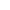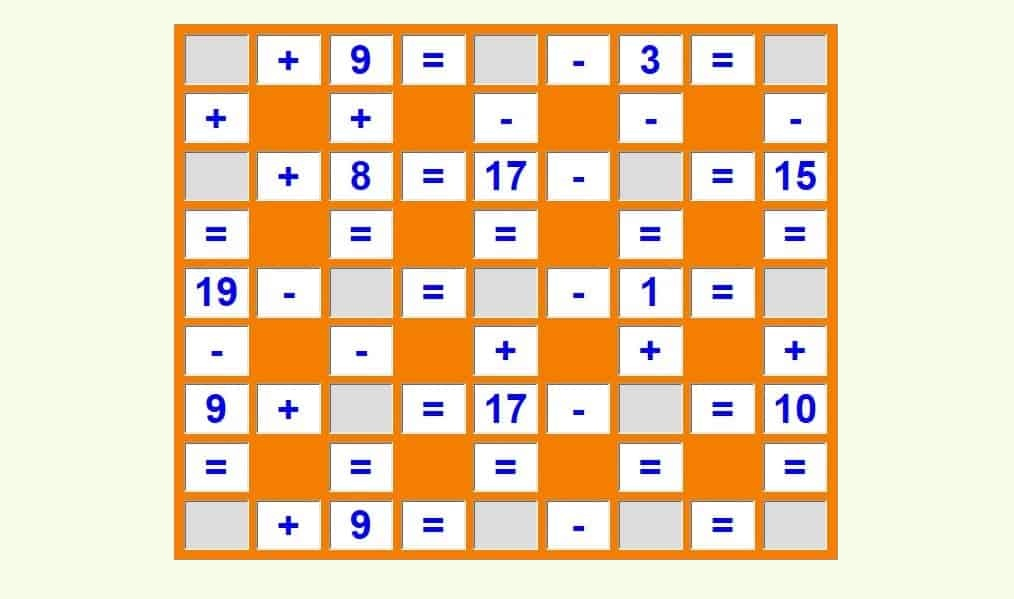﻿ Simple maths puzzles with answers: games and worksheets

simple maths puzzles with answers PDF. printable maths games and puzzles pdf. mathematical puzzles with answers pdf free download. 100 maths puzzles pdf. printable math puzzles PDF. addition and subtraction puzzles with answers. maths puzzles pdf. math puzzle games with answers.

New game# Simple maths puzzles with answers

Fill in the blanks so that each equation is correct. You can choose the maximum value: 20, 30, 50 or 100.

## Simple maths puzzles for kids and adultsPlay online maths puzzles games, check results, find mistakes and play again. Fill in the missing numbers so the operations are correct.

First, look for an operation that you can finish. With the number found you can solve another equation. Continue to do this until all operations are solved and the math puzzle is completed.

Press "Check it" to find out if the math puzzle is filled in correctly. The correct answers will be green, wrong results will be red. Press the button "New game" and the new simple math puzzle will be generated.

Each equation should be solved from left to right and from top to bottom in this math puzzle. If you are not sure how to solve these maths puzzles correctly, press "Check it" button first and you will see the answer key. You can also watch this video on YouTube.

Do you want to try something easier? Then play these online math crossword puzzles.

## 100 maths puzzles PDF

Help your kids to learn addition and subtraction easily. Make math more enjoyable with these awesome math puzzles. Students correct their errors by themselves.

Look inside

## Free printable maths puzzles with answers in PDF

Download free printable maths puzzles with answers in PDF below. The worksheet has two pages, the first one is the math puzzle and the other one page contains the answers.

## Why to play maths puzzles games?

To learn math online is fun. Thousands of new maths puzzles can be generated again and again, you don´t need to print any worksheet and you see immediately, where you made mistakes.

To play these math puzzle games you should already master addition up to 20 and subtraction within 20.

These simple maths puzzles teach addition and subtraction. Kids love this games, because they can learn math easily when they control their own learning. Read more about it in Psychology Today.